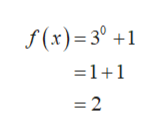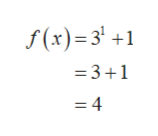Sketch the graph of f (x) = 3^x +1

Question

Sketch the graph of f (x) = 3^x +1

Step 1

The given function is,

Step 2

Substitute x=0  in equation 1.help_outlineImage Transcriptionclose- 3° +1 f(x) 30 - 1 +1 = 2 fullscreen
Step 3

Substitute x=1  ...help_outlineImage Transcriptionclose= 3 +1 =3 +1 = 4 fullscreen

Want to see the full answer?

See Solution

Want to see this answer and more?

Our solutions are written by experts, many with advanced degrees, and available 24/7

See Solution
Tagged in

Other